### 11. CONFIDENCE INTERVALS AND THEIR ARBITRARINESS

So far we have worked only in terms of relative probabilities and rms values to give an idea of the accuracy of the determination=*. One can also ask the question, What is the probability thatlies between two certain values such as' and"? This is called a confidence interval,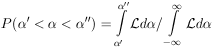Unfortunately such a probability depends on the arbitrary choice of what quantity is chosen for. To show this consider the area under the tail of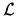().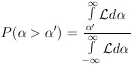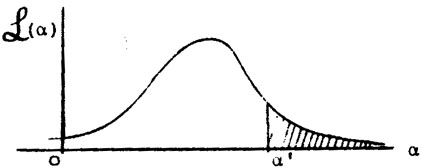Figure 3. Shaded area is P(>'). (Sometimes called the confidence limit of'.)

If=() had been chosen as the physical parameter instead, the same confidence interval is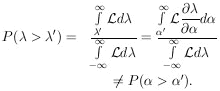Thus, in general, the numerical value of a confidence interval depends on the choice of the physical parameter. This is also true to some extent in evaluating. Only the maximum likelihood solution and the relative probabilities are unaffected by the choice of. For Gaussian distributions, confidence intervals can be evaluated by using tables of the probability integral. Tables of cumulative binomial distributions and cumulative Poisson distributions are also available. Appendix V contains a plot of the cumulative Gaussian distribution.